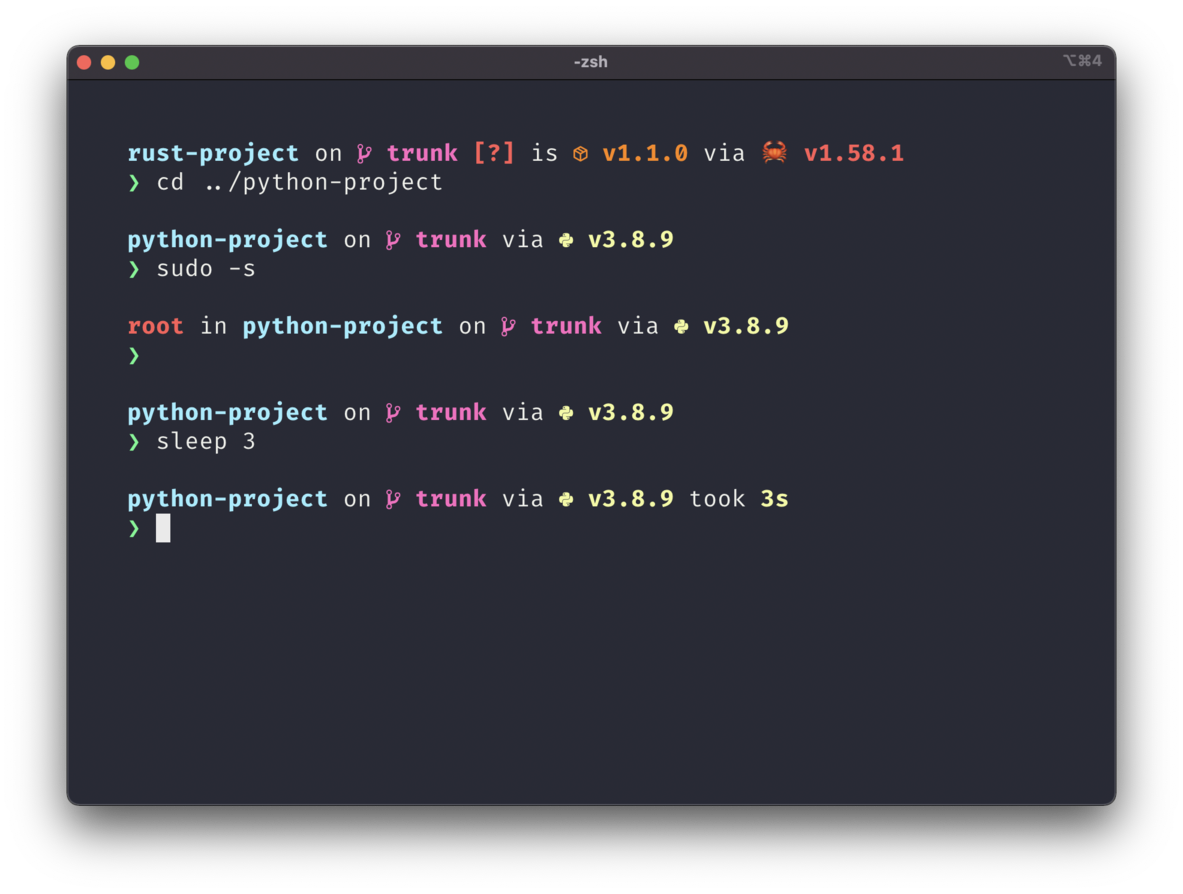# # Nerd Font Symbols Preset

This preset changes the symbols for each module to use Nerd Font symbols.### # Configuration

``````starship preset nerd-font-symbols -o ~/.config/starship.toml
``````

``````[aws]
symbol = "  "

[buf]
symbol = " "

[c]
symbol = " "

[conda]
symbol = " "

[dart]
symbol = " "

[directory]

[docker_context]
symbol = " "

[elixir]
symbol = " "

[elm]
symbol = " "

[fossil_branch]
symbol = " "

[git_branch]
symbol = " "

[golang]
symbol = " "

[guix_shell]
symbol = " "

symbol = " "

[haxe]
symbol = " "

[hg_branch]
symbol = " "

[hostname]
ssh_symbol = " "

[java]
symbol = " "

[julia]
symbol = " "

[lua]
symbol = " "

[memory_usage]
symbol = "󰍛 "

[meson]
symbol = "󰔷 "

[nim]
symbol = "󰆥 "

[nix_shell]
symbol = " "

[nodejs]
symbol = " "

[os.symbols]
Alpaquita = " "
Alpine = " "
Amazon = " "
Android = " "
Arch = " "
Artix = " "
CentOS = " "
Debian = " "
DragonFly = " "
Emscripten = " "
EndeavourOS = " "
Fedora = " "
FreeBSD = " "
Garuda = "󰛓 "
Gentoo = " "
HardenedBSD = "󰞌 "
Illumos = "󰈸 "
Linux = " "
Mabox = " "
Macos = " "
Manjaro = " "
Mariner = " "
MidnightBSD = " "
Mint = " "
NetBSD = " "
NixOS = " "
OpenBSD = "󰈺 "
openSUSE = " "
OracleLinux = "󰌷 "
Pop = " "
Raspbian = " "
Redhat = " "
RedHatEnterprise = " "
Redox = "󰀘 "
Solus = "󰠳 "
SUSE = " "
Ubuntu = " "
Unknown = " "
Windows = "󰍲 "

[package]
symbol = "󰏗 "

[pijul_channel]
symbol = " "

[python]
symbol = " "

[rlang]
symbol = "󰟔 "

[ruby]
symbol = " "

[rust]
symbol = " "

[scala]
symbol = " "
``````